Author: Oscar Cronquist Article last updated on March 31, 2023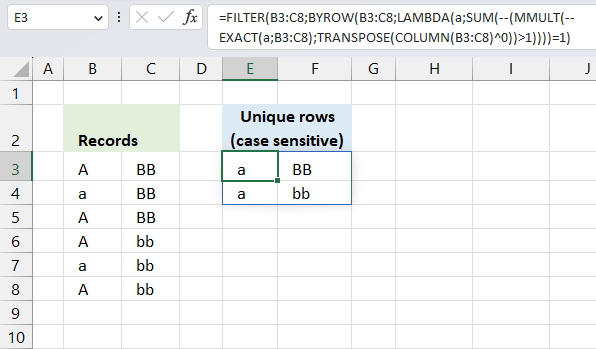This article demonstrates two ways to extract unique and unique distinct rows from a given cell range. The first one is an Excel 365 LAMBDA function, the second is a User Defined Function.

## 1. Filter unique rows case sensitive - Excel 365 LAMBDA functionThe following formula lists unique rows from cell range B3:C8 also considering upper and lower letters, in other words, a case sensitive list.

The formula extracts row 4 and row 7, they are the only ones that are unique meaning they exist only once in the list. The other rows have at least one duplicate.

Excel 365 formula in cell E3:

=FILTER(B3:C8,BYROW(B3:C8,LAMBDA(a,SUM(--(MMULT(--EXACT(a,B3:C8),TRANSPOSE(COLUMN(B3:C8)^0))>1))))=1)

### Explaining formula

#### Step 1 - Case sensitive compare

The EXACT function checks if two values are precisely the same, it returns TRUE or FALSE. The EXACT function also considers upper case and lower case letters.

Function syntax: EXACT(text1, text2)

EXACT(a,B3:C8)

Variable a is specified in the LAMBDA function in step 10, the LAMBDA function is used together with the BYROW function to perform calculations row-wise.

For example, the first row in cell range B3:C8 is B3:C3.

EXACT(B3:C3, B3:C8)

becomes

EXACT({"A","BB"},{"A","BB";"a","BB";"A","BB";"A","bb";"a","bb";"A","bb"})

and returns

{TRUE, TRUE;FALSE, TRUE;TRUE, TRUE;TRUE, FALSE;FALSE, FALSE;TRUE, FALSE}.

#### Step 2 - Convert boolean to numerical

The MMULT function in step 6 requires numerical values to work properly, there are several ways to convert boolean values to their numerical equivalents. One is to use double negatives, other examples are +0 (zero), *1.

--EXACT(a,B3:C8)

becomes

--{TRUE, TRUE;FALSE, TRUE;TRUE, TRUE;TRUE, FALSE;FALSE, FALSE;TRUE, FALSE}

and returns

{1, 1;0, 1;1, 1;1, 0;0, 0;1, 0}.

#### Step 3 - Calculate column numbers in given cell range

The COLUMN function returns the column number of the top-left cell of a cell reference.

Function syntax: COLUMN(reference)

COLUMN(B3:C8)

returns

{2, 3}

#### Step 4 - Raise array to the power of 0 (zero)

The ^ caret character represents an exponent, in this case, an array raised to the power of 0 (zero). This creates an array containing only 1's.

COLUMN(B3:C8)^0

becomes

{2, 3}^0

and returns

{1, 1}

#### Step 5 - Rearrange values from horizontal to vertical

The TRANSPOSE function converts a vertical range to a horizontal range, or vice versa.

Function syntax: TRANSPOSE(array)

TRANSPOSE(COLUMN(B3:C8)^0)

becomes

TRANSPOSE({1, 1})

and returns

{1; 1}

#### Step 6 - Perform matrix multiplication

The MMULT function calculates the matrix product of two arrays, an array as the same number of rows as array1 and columns as array2.

Function syntax: MMULT(array1, array2)

MMULT(--EXACT(a,B3:C8),TRANSPOSE(COLUMN(B3:C8)^0))

The following calculation is only for the first row, the formula calculates the remaining rows as well but not shown here.

MMULT(--EXACT(B3:C3,B3:C8),TRANSPOSE(COLUMN(B3:C8)^0))

becomes

MMULT({1,1;0,1;1,1;1,0;0,0;1,0},{1;1})

and returns

{2; 1; 2; 1; 0; 1}.

#### Step 7 - Check if numbers are larger than 1

The larger than character is a logical operator, it lets you check if a value is larger than another given value. The result is a boolean value TRUE or FALSE.

MMULT(--EXACT(a,B3:C8),TRANSPOSE(COLUMN(B3:C8)^0))>1

becomes

{2; 1; 2; 1; 0; 1}>1

and returns

{TRUE; FALSE; TRUE; FALSE; FALSE; FALSE}

#### Step 8 - Convert boolean to numerical

The SUM function can't process boolean values, we must once again convert the boolean values to their numerical equivalents using double negatives just like in step 2.

--(MMULT(--EXACT(a,B3:C8),TRANSPOSE(COLUMN(B3:C8)^0))>1)

becomes

--(MMULT(--EXACT(B3:C3,B3:C8),TRANSPOSE(COLUMN(B3:C8)^0))>1)

becomes

--{TRUE; FALSE; TRUE; FALSE; FALSE; FALSE}

and returns

{1; 0; 1; 0; 0; 0}.

This array tells us that the value in cell B3:C3 (first position in the array) is exactly the same as the value in the third position in the array. The position in the array corresponds to the position in cell range B3:C8.

#### Step 9 - Add numbers and return a total

The SUM function allows you to add numerical values, the function returns the sum in the cell it is entered in. The SUM function is cleverly designed to ignore text and boolean values, adding only numbers.

Function syntax: SUM(number1, [number2], ...)

SUM(--(MMULT(--EXACT(a,B3:C8),TRANSPOSE(COLUMN(B3:C8)^0))>1))

becomes

SUM({1; 0; 1; 0; 0; 0})

and returns 2. This means that the first row has a duplicate and its located on row 3. Number 1 in the array is also in the third position.

#### Step 10 - Build LAMBDA function

The LAMBDA function build custom functions without VBA, macros or javascript.

Function syntax: LAMBDA([parameter1, parameter2, …,] calculation)

LAMBDA(a,SUM(--(MMULT(--EXACT(a,B3:C8),TRANSPOSE(COLUMN(B3:C8)^0))>1)))

The LAMBDA function is needed for the BYROW function to work properly.

#### Step 11 - Perform calculations row-wise

The BYROW function puts values from an array into a LAMBDA function row-wise.

Function syntax: BYROW(array, lambda(array, calculation))

BYROW(B3:C8,LAMBDA(a,SUM(--(MMULT(--EXACT(a,B3:C8),TRANSPOSE(COLUMN(B3:C8)^0))>1))))

#### Step 12 - Filter rows if array is equal to 1

The FILTER function extracts values/rows based on a condition or criteria.

Function syntax: FILTER(array, include, [if_empty])

FILTER(B3:C8,BYROW(B3:C8,LAMBDA(a,SUM(--(MMULT(--EXACT(a,B3:C8),TRANSPOSE(COLUMN(B3:C8)^0))>1))))=1)

becomes

FILTER(B3:C8,{2;1;2;2;1;2}=1)

becomes

FILTER(B3:C8,{FALSE;TRUE;FALSE;FALSE;TRUE;FALSE})

and returns

{"a", "BB"; "a", "bb"}.

## 2. Count unique rows case sensitive - Excel 365 LAMBDA function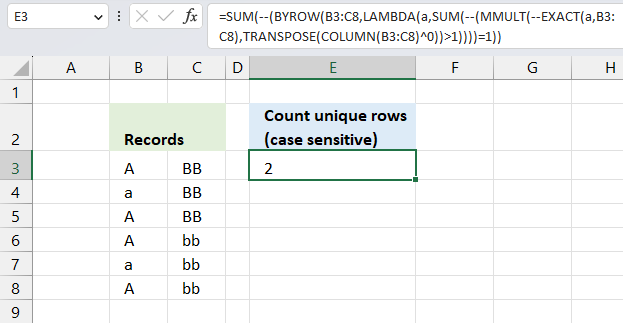The image above demonstrates an Excel 365 formula in cell F3 that calculates the number of unique rows but also considering upper and lower letters, specified in cell range C3:D8.

The unique rows are in B4:C4 and B7:C7, the remaining rows have at least one duplicate and is therefore not unique.

Excel 365 LAMBDA formula in cell F3:

=SUM(--(BYROW(B3:C8,LAMBDA(a,SUM(--(MMULT(--EXACT(a,B3:C8),TRANSPOSE(COLUMN(B3:C8)^0))>1))))=1))

### Explaining formula

#### Step 1 - Case sensitive compare

The EXACT function checks if two values are precisely the same, it returns TRUE or FALSE. The EXACT function also considers upper case and lower case letters.

Function syntax: EXACT(text1, text2)

EXACT(a,B3:C8)

Variable a is specified in the LAMBDA function in step 10, the LAMBDA function is used together with the BYROW function to perform calculations row-wise.

For example, the first row in cell range B3:C8 is B3:C3.

EXACT(B3:C3, B3:C8)

becomes

EXACT({"A","BB"},{"A","BB";"a","BB";"A","BB";"A","bb";"a","bb";"A","bb"})

and returns

{TRUE, TRUE;FALSE, TRUE;TRUE, TRUE;TRUE, FALSE;FALSE, FALSE;TRUE, FALSE}.

#### Step 2 - Convert boolean to numerical

The MMULT function in step 6 requires numerical values to work properly, there are several ways to convert boolean values to their numerical equivalents. One is to use double negatives, other examples are +0 (zero), *1.

--EXACT(a,B3:C8)

becomes

--{TRUE, TRUE;FALSE, TRUE;TRUE, TRUE;TRUE, FALSE;FALSE, FALSE;TRUE, FALSE}

and returns

{1, 1;0, 1;1, 1;1, 0;0, 0;1, 0}.

#### Step 3 - Calculate column numbers in given cell range

The COLUMN function returns the column number of the top-left cell of a cell reference.

Function syntax: COLUMN(reference)

COLUMN(B3:C8)

returns

{2, 3}

#### Step 4 - Raise array to the power of 0 (zero)

The ^ caret character represents an exponent, in this case, an array raised to the power of 0 (zero). This creates an array containing only 1's.

COLUMN(B3:C8)^0

becomes

{2, 3}^0

and returns

{1, 1}

#### Step 5 - Rearrange values from horizontal to vertical

The TRANSPOSE function converts a vertical range to a horizontal range, or vice versa.

Function syntax: TRANSPOSE(array)

TRANSPOSE(COLUMN(B3:C8)^0)

becomes

TRANSPOSE({1, 1})

and returns

{1; 1}

#### Step 6 - Perform matrix multiplication

The MMULT function calculates the matrix product of two arrays, an array as the same number of rows as array1 and columns as array2.

Function syntax: MMULT(array1, array2)

MMULT(--EXACT(a,B3:C8),TRANSPOSE(COLUMN(B3:C8)^0))

The following calculation is only for the first row, the formula calculates the remaining rows as well but not shown here.

MMULT(--EXACT(B3:C3,B3:C8),TRANSPOSE(COLUMN(B3:C8)^0))

becomes

MMULT({1,1;0,1;1,1;1,0;0,0;1,0},{1;1})

and returns

{2; 1; 2; 1; 0; 1}.

#### Step 7 - Check if numbers are larger than 1

The larger than character is a logical operator, it lets you check if a value is larger than another given value. The result is a boolean value TRUE or FALSE.

MMULT(--EXACT(a,B3:C8),TRANSPOSE(COLUMN(B3:C8)^0))>1

becomes

{2; 1; 2; 1; 0; 1}>1

and returns

{TRUE; FALSE; TRUE; FALSE; FALSE; FALSE}.

Remember that the above array calculation is only for B3:C3, the remaining rows will also be calculated , however, not shown here.

#### Step 8 - Convert boolean to numerical

The SUM function can't process boolean values, we must once again convert the boolean values to their numerical equivalents using double negatives just like in step 2.

--(MMULT(--EXACT(a,B3:C8),TRANSPOSE(COLUMN(B3:C8)^0))>1)

becomes

--(MMULT(--EXACT(B3:C3,B3:C8),TRANSPOSE(COLUMN(B3:C8)^0))>1)

becomes

--{TRUE;FALSE;TRUE;FALSE;FALSE;FALSE}

and returns

{1; 0; 1; 0; 0; 0}.

This array tells us that the value in cell B3:C3 (first position in the array) is exactly the same as the value in the third position in the array. The position in the array corresponds to the position in cell range B3:C8.

#### Step 9 - Add numbers and return a total

The SUM function allows you to add numerical values, the function returns the sum in the cell it is entered in. The SUM function is cleverly designed to ignore text and boolean values, adding only numbers.

Function syntax: SUM(number1, [number2], ...)

SUM(--(MMULT(--EXACT(a,B3:C8),TRANSPOSE(COLUMN(B3:C8)^0))=1))

becomes

SUM({1; 0; 1; 0; 0; 0})

and returns 2. This means that the first row has a duplicate and its located on row 3. Number 1 in the array is also in the third position.

#### Step 10 - Build LAMBDA function

The LAMBDA function build custom functions without VBA, macros or javascript.

Function syntax: LAMBDA([parameter1, parameter2, …,] calculation)

LAMBDA(a,SUM(--(MMULT(--EXACT(a,B3:C8),TRANSPOSE(COLUMN(B3:C8)^0))=1)))

The LAMBDA function is needed for the BYROW function to work properly.

#### Step 11 - Perform calculations row-wise

The BYROW function puts values from an array into a LAMBDA function row-wise.

Function syntax: BYROW(array, lambda(array, calculation))

BYROW(B3:C8,LAMBDA(a,SUM(--(MMULT(--EXACT(a,B3:C8),TRANSPOSE(COLUMN(B3:C8)^0))=1))))

returns

{2; 1; 2; 2; 1; 2}.

This array shows the calculation for each row. B3:C3 is the first row, it returned 2 which is the first value in the array. B4:C4 returns 1 which is the second value in the array, and so on.

#### Step 12 - Compare with 1

The equal sign lets you compare values in an Excel formula, it is a logical operator and returns TRUE or FALSE. By comparing the array with 1 we can celculate the number of unique rows there is in cell range B3:C8.

--(BYROW(B3:C8,LAMBDA(a,SUM(--(MMULT(--EXACT(a,B3:C8),TRANSPOSE(COLUMN(B3:C8)^0))>1))))=1)

becomes

--({2; 1; 2; 2; 1; 2}=1)

becomes

--({FALSE; TRUE; FALSE; FALSE; TRUE; FALSE})

and returns

{0; 1; 0; 0; 1; 0}.

The SUM function allows you to add numerical values, the function returns the sum in the cell it is entered in. The SUM function is cleverly designed to ignore text and boolean values, adding only numbers.

Function syntax: SUM(number1, [number2], ...)

SUM(--(BYROW(B3:C8,LAMBDA(a,SUM(--(MMULT(--EXACT(a,B3:C8),TRANSPOSE(COLUMN(B3:C8)^0))>1))))=1))

becomes

SUM({0; 1; 0; 0; 1; 0})

and returns 2. There are two unique rows, also considering upper and lower letters, in cell range C3:D8.

## 3. Filter unique distinct rows case sensitive - Excel 365 recursive LAMBDA function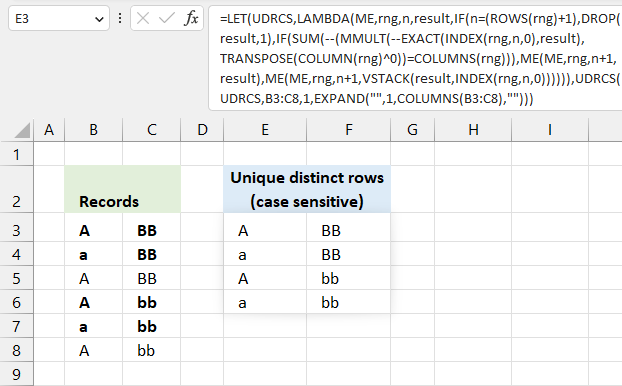The following formula extracts unique distinct rows case sensitive from a given cell range, the formula is dynamic meaning it takes into account the number of columns as well.  This means that the formula also works for cell ranges larger than two columns.

If your cell range only has one column then use this much smaller formula: Extract a case sensitive unique list from a column - Excel 365

How to use the formula? Change cell reference B3:C8 to your needs, there are two instances of B3:C8 that need to be changed in the formula below.

Excel 365 recursive LAMBDA formula in cell E3:

=LET(UDRCS,LAMBDA(ME,rng,n,result,
IF(n=(ROWS(rng)+1),DROP(result,1),
IF(SUM(--(MMULT(--EXACT(INDEX(rng,n,0),result),TRANSPOSE(COLUMN(rng)^0))=COLUMNS(rng))),
ME(ME,rng,n+1,result),ME(ME,rng,n+1,VSTACK(result,INDEX(rng,n,0))))))
,UDRCS(UDRCS,B3:C8,1,EXPAND("",1,COLUMNS(B3:C8),"")))

## 4. Count unique distinct rows case sensitive - Excel 365 LAMBDA function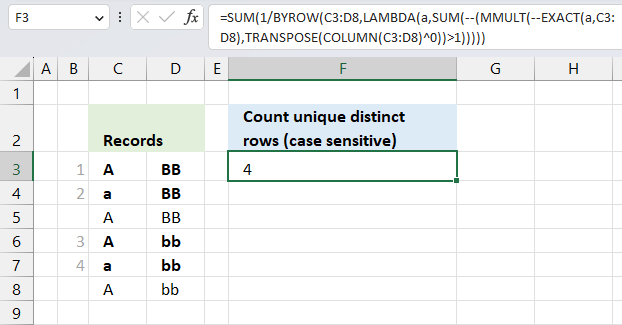The Excel 365 formula in cell F3 shown in the image above calculates the number of unqiue distinct rows, also considering upper and lower letters, specified in cell range C3:D8.

I have bolded, and put numbers next to, unique distinct rows in the image above.

Excel 365 LAMBDA formula in cell F3:

=SUM(1/BYROW(C3:D8,LAMBDA(a,SUM(--(MMULT(--EXACT(a,C3:D8),TRANSPOSE(COLUMN(C3:D8)^0))>1)))))

### Explaining formula

#### Step 1 - Case sensitive compare

The EXACT function checks if two values are precisely the same, it returns TRUE or FALSE. The EXACT function also considers upper case and lower case letters.

Function syntax: EXACT(text1, text2)

EXACT(a,B3:C8)

Variable a is specified in the LAMBDA function in step 10, the LAMBDA function is used together with the BYROW function to perform calculations row-wise.

For example, the first row in cell range B3:C8 is B3:C3.

EXACT(B3:C3, B3:C8)

becomes

EXACT({"A","BB"},{"A","BB";"a","BB";"A","BB";"A","bb";"a","bb";"A","bb"})

and returns

{TRUE, TRUE;FALSE, TRUE;TRUE, TRUE;TRUE, FALSE;FALSE, FALSE;TRUE, FALSE}.

#### Step 2 - Convert boolean to numerical

The MMULT function in step 6 requires numerical values to work properly, there are several ways to convert boolean values to their numerical equivalents. One is to use double negatives, other examples are +0 (zero), *1.

--EXACT(a,B3:C8)

becomes

--{TRUE, TRUE;FALSE, TRUE;TRUE, TRUE;TRUE, FALSE;FALSE, FALSE;TRUE, FALSE}

and returns

{1, 1;0, 1;1, 1;1, 0;0, 0;1, 0}.

#### Step 3 - Calculate column numbers in given cell range

The COLUMN function returns the column number of the top-left cell of a cell reference.

Function syntax: COLUMN(reference)

COLUMN(B3:C8)

returns

{2, 3}

#### Step 4 - Raise array to the power of 0 (zero)

The ^ caret character represents an exponent, in this case, an array raised to the power of 0 (zero). This creates an array containing only 1's.

COLUMN(B3:C8)^0

becomes

{2, 3}^0

and returns

{1, 1}

#### Step 5 - Rearrange values from horizontal to vertical

The TRANSPOSE function converts a vertical range to a horizontal range, or vice versa.

Function syntax: TRANSPOSE(array)

TRANSPOSE(COLUMN(B3:C8)^0)

becomes

TRANSPOSE({1, 1})

and returns

{1; 1}

#### Step 6 - Perform matrix multiplication

The MMULT function calculates the matrix product of two arrays, an array as the same number of rows as array1 and columns as array2.

Function syntax: MMULT(array1, array2)

MMULT(--EXACT(a,B3:C8),TRANSPOSE(COLUMN(B3:C8)^0))

The following calculation is only for the first row, the formula calculates the remaining rows as well but not shown here.

MMULT(--EXACT(B3:C3,B3:C8),TRANSPOSE(COLUMN(B3:C8)^0))

becomes

MMULT({1,1;0,1;1,1;1,0;0,0;1,0},{1;1})

and returns

{2; 1; 2; 1; 0; 1}.

#### Step 7 - Check if numbers are larger than 1

The larger than character is a logical operator, it lets you check if a value is larger than another given value. The result is a boolean value TRUE or FALSE.

MMULT(--EXACT(a,B3:C8),TRANSPOSE(COLUMN(B3:C8)^0))>1

becomes

{2; 1; 2; 1; 0; 1}>1

and returns

{TRUE; FALSE; TRUE; FALSE; FALSE; FALSE}

#### Step 8 - Convert boolean to numerical

The SUM function can't process boolean values, we must once again convert the boolean values to their numerical equivalents using double negatives just like in step 2.

--(MMULT(--EXACT(a,B3:C8),TRANSPOSE(COLUMN(B3:C8)^0))>1)

becomes

--(MMULT(--EXACT(B3:C3,B3:C8),TRANSPOSE(COLUMN(B3:C8)^0))>1)

becomes

--{TRUE; FALSE; TRUE; FALSE; FALSE; FALSE}

and returns

{1; 0; 1; 0; 0; 0}.

This array tells us that the value in cell B3:C3 (first position in the array) is exactly the same as the value in the third position in the array. The position in the array corresponds to the position in cell range B3:C8.

#### Step 9 - Add numbers and return a total

The SUM function allows you to add numerical values, the function returns the sum in the cell it is entered in. The SUM function is cleverly designed to ignore text and boolean values, adding only numbers.

Function syntax: SUM(number1, [number2], ...)

SUM(--(MMULT(--EXACT(a,B3:C8),TRANSPOSE(COLUMN(B3:C8)^0))>1))

becomes

SUM({1; 0; 1; 0; 0; 0})

and returns 2. This means that the first row has a duplicate and its located on row 3. Number 1 in the array is also in the third position.

#### Step 10 - Build LAMBDA function

The LAMBDA function build custom functions without VBA, macros or javascript.

Function syntax: LAMBDA([parameter1, parameter2, …,] calculation)

LAMBDA(a,SUM(--(MMULT(--EXACT(a,B3:C8),TRANSPOSE(COLUMN(B3:C8)^0))>1)))

The LAMBDA function is needed for the BYROW function to work properly.

#### Step 11 - Perform calculations row-wise

The BYROW function puts values from an array into a LAMBDA function row-wise.

Function syntax: BYROW(array, lambda(array, calculation))

BYROW(B3:C8,LAMBDA(a,SUM(--(MMULT(--EXACT(a,B3:C8),TRANSPOSE(COLUMN(B3:C8)^0))>1))))

#### Step 12 - Divide 1 by all numbers in the array

The division character lets you dived numbers in an Excel formula.

1/BYROW(C3:D8,LAMBDA(a,SUM(--(MMULT(--EXACT(a,C3:D8),TRANSPOSE(COLUMN(C3:D8)^0))>1))))

becomes

1/{2; 1; 2; 2; 1; 2}

and returns

{0.5; 1; 0.5; 0.5; 1; 0.5}.

The SUM function allows you to add numerical values, the function returns the sum in the cell it is entered in. The SUM function is cleverly designed to ignore text and boolean values, adding only numbers.

Function syntax: SUM(number1, [number2], ...)

SUM(1/BYROW(C3:D8,LAMBDA(a,SUM(--(MMULT(--EXACT(a,C3:D8),TRANSPOSE(COLUMN(C3:D8)^0))>1)))))

becomes

SUM({0.5; 1; 0.5; 0.5; 1; 0.5})

and returns 4. There are four unique distinct rows, also considering upper and lower letters, in cell range C3:D8.

## 5. Filter unique distinct records case sensitive - UDF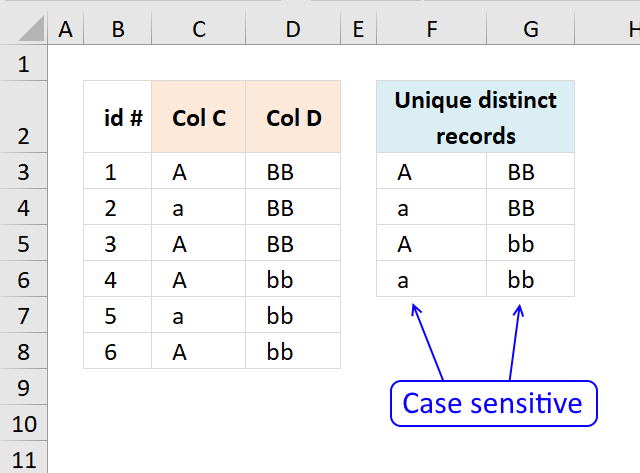The User Defined Function demonstrated above extracts unique distinct records also considering upper and lower case letters. For example, a record containing  A and B is not the same as a record containing a and b.

A User defined Function is a custom function anyone can build, you simply add the VBA code to your workbook and you are good to go.

Array formula in cell F3:G6:

=UniqueRecords(C3:D8)

To enter an array formula, type the formula in a cell then press and hold CTRL + SHIFT simultaneously, now press Enter once. Release all keys.

The formula bar now shows the formula with a beginning and ending curly bracket telling you that you entered the formula successfully. Don't enter the curly brackets yourself.

### User defined Function Syntax

UniqueRecords(rng)

### Arguments

 Parameter Text rng Required. The range you want to use.

### Where to copy VBA code?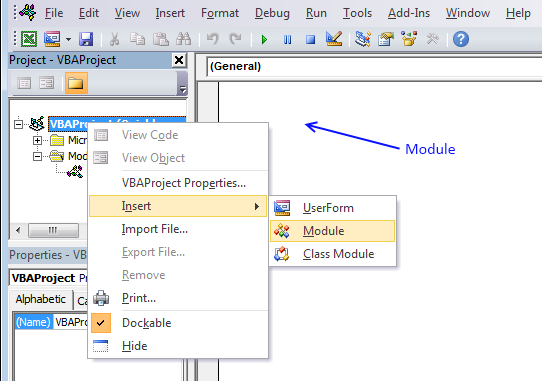1. Press Alt-F11 to open visual basic editor
2. Press with left mouse button on Module on the Insert menu
3. Copy and paste above vba code
4. Exit visual basic editor

### Excel user defined function:

```'Name function and declare argument
Function UniqueRecords(rng As Variant) As Variant()
' This udf filters unique distinct records (case sensitive)
'Declare variables and data types
Dim r As Single, c As Single, temp() As Variant, k As Single
Dim rt As Single, ct As Single, a As Single, b As Single
Dim i As Single, j As Integer, iCols As Single, iRows As Single

'Save values in cell range to array variable rng (yes, same name)
rng = rng.Value
'Change size of array variable rng
ReDim temp(UBound(rng, 2) - 1, 0)
'Iterate through rows in array variable rng
For r = 1 To UBound(rng, 1)
'Iterate through rows in array variable temp
For rt = LBound(temp, 2) To UBound(temp, 2)
'Iterate through columns in array variable rng
For c = 1 To UBound(rng, 2)
' If temp value is equal rng value then increment variable a with 1
If temp(c - 1, rt) = rng(r, c) Then a = a + 1
Next c
'If a is equal to the number of columns in rng then all values in record match
If a = UBound(rng, 2) Then
a = 0
b = 0
Exit For
Else
a = 0
b = b + 1
End If
Next rt
If b = UBound(temp, 2) + 1 Then
For c = 1 To UBound(rng, 2)
temp(c - 1, UBound(temp, 2)) = rng(r, c)
Next c
ReDim Preserve temp(UBound(temp, 1), UBound(temp, 2) + 1)
b = 0
End If
Next r

If Range(Application.Caller.Address).Columns.Count < UBound(rng, 2) Then
MsgBox "There are more columns, extend user defined function to the right"
End If
If k < UBound(temp, 2) Then
MsgBox "There are more rows, extend user defined function down"
Else
For i = UBound(temp, 2) To k
ReDim Preserve temp(UBound(temp, 1), i)
For j = 0 To UBound(temp, 1)
temp(j, i) = ""
Next j
Next i
End If

UniqueRecords = Application.Transpose(temp)
End Function
```

### Get the Excel fileunqiue-distinct-records-case-sensitive.xls